# 20th LSI Design Contests・in Okinawa  Design Specification - 3-1

## 3-1. HOG

Histogram of Oriented Gradients (HOG) is a feature descriptor used in computer vision and image processing for the purpose of object detection. We can get quantity of strong characteristic from the shape change of the object by dividing a local domain into plural blocks, and making the incline of each the histogram.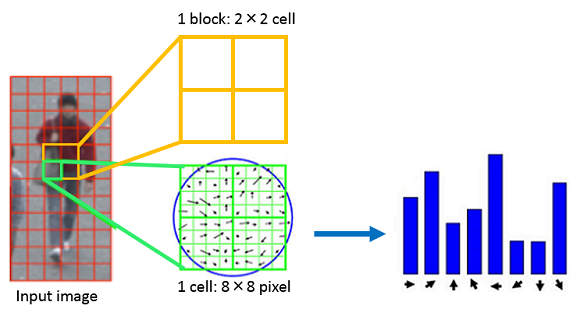Fig 1：A flow until becoming histogram

・The following (1) - (5) is the process to get quantity of HOG.

(1) Resize an image to pixel size and read it in gray scale.

(2) Calculate gradient magnitude and gradient angle from the brightness of each pixel.

We calculated gradient magnitude and the gradient angle by the following expressions.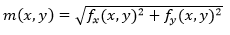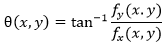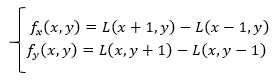(3) Calculate the histogram of gradient in each cell.

We quantized gradient angle each 20 degrees from zero degree to 180 degrees in 9 directions and calculate histogram in each cell domain.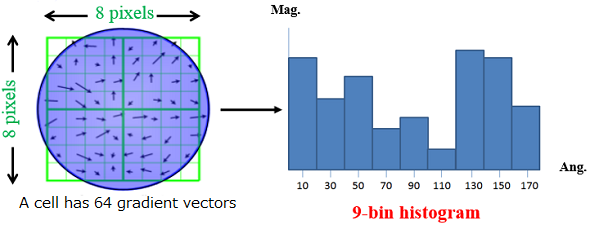Fig 2：Histogram of 9 directions

(4) Normalize each block.

We normalized each block by the following expressions.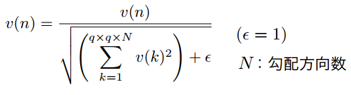v(n) is gradient angle histogram. q is cell size. N is the number of gradient angle. In addition, the denominator is a grand total of the quantity of HOG characteristic included in 1 block (q X q cell). For one cell, We normalize multiple times.

(5) Integrate all histogram.